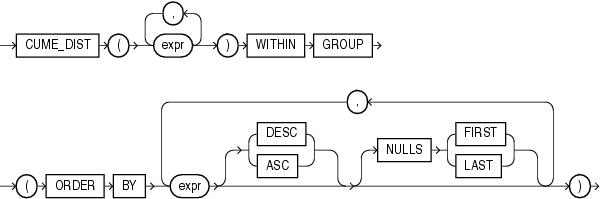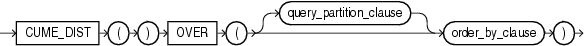# CUME_DIST

Aggregate Syntax

cume_dist_aggregate::=Description of the illustration cume_dist_aggregate.gif

Analytic Syntax

cume_dist_analytic::=Description of the illustration cume_dist_analytic.gif

"Analytic Functions" for information on syntax, semantics, and restrictions

Purpose

`CUME_DIST` calculates the cumulative distribution of a value in a group of values. The range of values returned by `CUME_DIST` is >0 to <=1. Tie values always evaluate to the same cumulative distribution value.

This function takes as arguments any numeric datatype or any nonnumeric datatype that can be implicitly converted to a numeric datatype. Oracle Database determines the argument with the highest numeric precedence, implicitly converts the remaining arguments to that datatype, makes the calculation, and returns `NUMBER`.

Table 2-10, "Implicit Type Conversion Matrix" for more information on implicit conversion and "Numeric Precedence" for information on numeric precedence
• As an aggregate function, `CUME_DIST` calculates, for a hypothetical row `r` identified by the arguments of the function and a corresponding sort specification, the relative position of row `r` among the rows in the aggregation group. Oracle makes this calculation as if the hypothetical row `r` were inserted into the group of rows to be aggregated over. The arguments of the function identify a single hypothetical row within each aggregate group. Therefore, they must all evaluate to constant expressions within each aggregate group. The constant argument expressions and the expressions in the `ORDER` `BY` clause of the aggregate match by position. Therefore, the number of arguments must be the same and their types must be compatible.

• As an analytic function, `CUME_DIST` computes the relative position of a specified value in a group of values. For a row `r`, assuming ascending ordering, the `CUME_DIST` of `r` is the number of rows with values lower than or equal to the value of `r`, divided by the number of rows being evaluated (the entire query result set or a partition).

Aggregate Example

The following example calculates the cumulative distribution of a hypothetical employee with a salary of \$15,500 and commission rate of 5% among the employees in the sample table `oe.employees`:

```SELECT CUME_DIST(15500, .05) WITHIN GROUP
(ORDER BY salary, commission_pct) "Cume-Dist of 15500"
FROM employees;

Cume-Dist of 15500
------------------
.972222222
```

Analytic Example

The following example calculates the salary percentile for each employee in the purchasing division. For example, 40% of clerks have salaries less than or equal to Himuro.

```SELECT job_id, last_name, salary, CUME_DIST()
OVER (PARTITION BY job_id ORDER BY salary) AS cume_dist
FROM employees
WHERE job_id LIKE 'PU%'
ORDER BY job_id, last_name, salary, cume_dist;

JOB_ID     LAST_NAME                     SALARY  CUME_DIST
---------- ------------------------- ---------- ----------
PU_CLERK   Baida                           2900         .8
PU_CLERK   Colmenares                      2500         .2
PU_CLERK   Himuro                          2600         .4
PU_CLERK   Khoo                            3100          1
PU_CLERK   Tobias                          2800         .6
PU_MAN     Raphaely                       11000          1
```